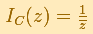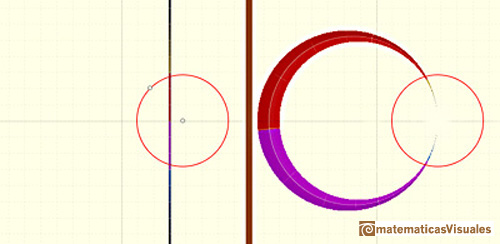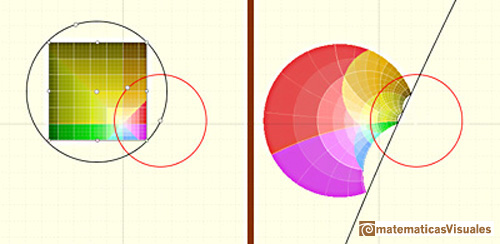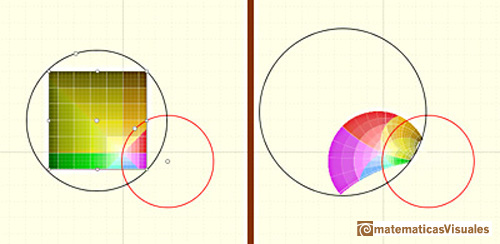Inversion is a one-to-one transformation of the points of the plane (except one point) by means of a given circle (circle of inversion). We shall call this circle C, of radius r and centre q. The center of the circle of inversion (center of inversion) has no image.

If the center of the circle of inversion is the origin and the radius is 1 this transformation has a simple formula:In the general case, this mapping is given by:The points on the circle of inversion transform into themselves and each point inside the circle is transformed to a point outside the circle (and vice-versa). Each point, its image and the center of the circle of inversion are in the same line. Sometimes we call this transformation as reflection in a circle.

Each line through the center of inversion is transformed in the same line.Each line not passing through the center of inversion is transformed in a circle through the center of inversion.The inverse of a circle is a straight line or a circle. It is a line if the circle passes through the center of inversion.The image of a circle not passing through the center of inversion is another circle not passing through the center of inversion.REFERENCES

Hilber and Cohn-Vossen - Geometry and the Imagination (pag. 253) - Chelsea Publishing Company
Coxeter - Introduction to Geometry - Wiley and Sons.
Pedoe - Circles, a Mathematical View - Dover
Tristan Needham - Visual Complex Analysis. (pag. 124) - Oxford University Press
Rademacher and Toeplitz - The Enjoyment of Mathematics
Ogilvy - Excursions in Geometry (pag. 24)- Oxford University PressInversion preserves the magnitud of angles but the sense is reversed. Orthogonal circles are mapped into orthogonal circlesThe Complex Cosine Function extends the Real Cosine Function to the complex plane. It is a periodic function that shares several properties with his real ancestor.The Complex Cosine Function maps horizontal lines to confocal ellipses.Una primera aproximación a estas transformaciones. Representación de dos haces coaxiales de circunferencias ortogonales.The Complex Exponential Function extends the Real Exponential Function to the complex plane.The usual definition of a function is restrictive. We may broaden the definition of a function to allow f(z) to have many differente values for a single value of z. In this case f is called a many-valued function or a multifunction.Examples of complex functions: polynomials, rationals and Moebius Transformations.